# CUET Physics Sample Paper

Common University Entrance Test for the admission in UG Courses CUET (UG) Physics Sample Paper for online mock practice of exam. The Physics is one of the science group subject in Section II of Test.

## CUET (UG) Sample Paper : Physics

Questions : attempt any 40 Questions out of 50
Time : 45 Minutes

Q.1: If point charges Q1 = 2×10-7C and Q2=3×10-7C are at 30 cm separation, then find electrostatic force between them.
(a) 2 x 10-3 N
(b) 6 x 10-3 N
(c) 5 x 10-3 N
(d) 1 x 10-3 N

Ans : (b) 6 x 10-3 N

Q.2: If two bodies are rubbed and one of them acquires q1 charge and another acquires q2 charge, then ratio of q1 : q2 is
(a) 1 : 2
(b) 2 : 1
(c) – 1 : 1
(d) 1 : 4

Ans : (c) – 1 : 1

Q.3: Two field lines can never cross each other because
(a) field lines are closed curves
(b) field lines repels each other
(c) field lines crowded only near the charge
(d) field has a unique direction at each point

Ans : (d) field has a unique direction at each point

Q.4: The potential difference between a cloud and the earth is 107 V. Calculate the amount of energy dissipated when the charge of 100 C is transferred from the cloud to the ground due to lightning bolt.
(a) zero
(b) 109 J
(c) 60 J
(d) 107 J

Ans : (b) 109 J

Q.5: Two charges 3 x 10-8C and -2 x 10-8 C located 15 cm apart. At what point on the line joining the two charges is the electric potential zero?
(a) 9 cm
(b) 42 cm
(c) 18 cm
(d) 20 cm

Ans : (a) 9 cm

Q.6: What is the work done in moving a 2µC point charge from corner A to corner B of a square ABCD in figure, when a 10µC charge exists at the centre of the square?

(a) Zero
(b) 5
(c) 2
(d) 20

Ans : (a) Zero

Q.7: A wire of resistance 4Ω is stretched to twice its original length. The resistance of stretched wire would be
(a) 2 Ω
(b) 4 Ω
(c) 8 Ω
(d) 16 Ω

Ans : (d) 16 Ω

Q.8: Kirchhoff’s junction rule is a reflection of
(a) conservation of current density vector
(b) conservation of charge
(c) the fact that the momentum with which a charged particle approaches a junction is unchanged (as a vector) as the charged particle leaves the junction
(d) the fact that there is a net accumulation of charges at a junction

Ans : (b) conservation of charge

Q.9: Determine the current drawn from source for the network shown below

(a)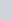$\frac{4}{17}$ A
(b)$\frac{10}{17}$ A
(c)$\frac{6}{17}$ A
(d)$\frac{5}{17}$ A

Ans : (b)$\frac{10}{17}$ A

Q.10: A deuteron of kinetic energy 50 keV is describing a circular orbit of radius 0.5 m in a plane perpendicular to magnetic field B. The kinetic energy of the proton that describes a circular orbit of radius 0.5 m in the same plane with the same magnetic field B is
(a) 25 keV
(b) 50 keV
(c) 200 keV
(d) 100 keV

Ans : (d) 100 keV

Q.11: A moving coil galvanometer is an instrument which
(a) is used to measure emf of cell
(b) is used to measure potential difference
(c) is used to measure resistance
(d) is a deflection type instrument that gives a deflection when a current flows through its coil

Ans : (d) is a deflection type instrument that gives a deflection when a current flows through its coil

Q.12: A circular coil of wire consisting of 100 turns, each of radius 8.0 cm carries a current of 0.40 A. What is the magnitude of the magnetic field B at the centre of the coil?
(a) 4 T
(b) 31 x 10-4 T
(c) 2 x 10-3T
(d) 10-4T

Ans : (b) 31 x 10-4 T

Q.13: A natural bar magnet
(a) is always suspended in north-south direction
(b) always has two poles
(c) is made of iron and its alloys
(d) All of the above

Ans : (d) All of the above

Q.14: At a certain place, horizontal component is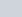$\sqrt3$ times the vertical component. The angle of dip at this place is
(a) zero
(b)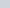$\pi$/3
(c)$\pi$/6
(d) None of these

Ans : (c)$\pi$/6

Q.15: A magnet of dipole moment m is aligned in equilibrium position in a magnetic field of intensity B. The work done to rotate it through an angle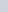$\theta$ in the magnetic field is
(a) mB sin$\theta$
(b) mB cos$\theta$
(c) mB (1 – cos$\theta$)
(d) mB (1 – sin$\theta$)

Ans : (c) mB (1 – cos$\theta$)

Q.16: Current in the coil is larger, when the magnet is

(a) pushed towards the coil faster
(b) pulled away from the coil faster
(c) Both (a) and (b)
(d) Neither (a) nor (b)

Ans : (c) Both (a) and (b)

Q.17: In induction furnace, heat is produced due to
(a) eddy current
(b) resistance
(c) capacitor
(d) None of these

Ans : (a) eddy current

Q.18: The mutual inductance M12 of coil 1 with respect to coil 2
(a) increases when they are brought nearer
(b) depends on the current passing through the coils
(c) increases when one of them is rotated about an axis
(d) is different from M21 of coil 2 with respect to coil 1

Ans : (a) increases when they are brought nearer

Q.19: If an AC main supply is given to be 220 V. What would be the average emf during a positive half-cycle?
(a) 198 V
(b) 386 V
(c) 256 V
(d) None of these

Ans : (a) 198 V

Q.20: If the frequency is doubled, what happen to the capacitive reactance and the current?
(a) Capacitive reactance is halved, the current is doubled
(b) Capacitive reactance is doubled, the current is halved
(c) Capacitive reactance and the current are halved
(d) Capacitive reactance and the current are doubled

Ans : (a) Capacitive reactance is halved, the current is doubled

Q.21: Which of the following current changes continuously in magnitude and periodically in direction?
(a) Direct current
(b) Induced current
(c) Alternating current
(d) Displacement current

Ans : (c) Alternating current

Q.22: Out of the following options, which one can be used to produce a propagating electromagnetic wave?
(a) A stationary charge
(b) A chargeless particle
(c) An accelerating charge
(d) A charge moving at constant velocity

Ans : (c) An accelerating charge

Q.23: The electric field produced by the radiation coming from a 100 W bulb at a distance of 3m is (assume that the efficiency of the bulb is 2.5% and it is a point source.)
(a) 4.5 V/m
(b) 2.8 V/m
(c) 5 V/m
(d) 4.07 V/m

Ans : (d) 4.07 V/m

Q.24: In an electromagnetic wave in free space, the root mean square value of the electric field is Erms = 6 V/m. The peak value of the magnetic field is
(a) 1.41 x 10-8 T
(b) 2.83 x 10-8 T
(c) 0.70 x 10-8 T
(d) 4.23 10-8 T

Ans : (b) 2.83 x 10-8 T

Q.25: A ray of light gets reflected from the plane mirror. The incident ray and the reflected ray are perpendicular to each other. The angle of incidence is
(a) 60°
(b) 30°
(c) 45°
(d) 0°

Ans : (c) 45°

Q.26: The nearer point of hypermetropic eye is 20 cm. Find the power of lens which can be used for its correction.
(a) + 2.5 D
(b) + 5.0 D
(c) – 1.5 D
(d) + 1.5 D

Ans : (b) + 5.0 D

Q.27: When a light ray enters from oil to glass, then on oil-glass interface, the velocity of light changes by a factor of [given, noil =2, nglass = 3/2 ]
(a)$\frac43$
(b)$\frac34$
(c) 3
(d) 1

Ans : (a)$\frac43$

Q.28: Two coherent sources of light can be obtained by
(a) two different lamps
(b) two different lamps but of the same power
(c) two different lamps of same power and having the same colours
(d) None of the above

Ans : (d) None of the above

Q.29: If a source is at infinity, then wavefronts reaching to observer are
(a) cylindrical
(b) spherical
(c) plane
(d) conical

Ans : (c) plane

Q.30: If the amplitude ratio of two sources producing interference is 3 : 5, then the ratio of intensities produced by each is
(a) 3 : 5
(b) 5 : 3
(c) 9 : 25
(d) 25 : 9

Ans : (c) 9 : 25

Q.31: Which of the following can be used to control the
intensity, in sunglasses, window-panes etc?
(a) Transverse wave
(b) Polaroids
(c) Plane polarised wave
(d) Polarised wave

Ans : (b) Polaroids

Q.32: The specific charge of a proton is 9.6 x 107 C kg-1. The specific charge of an a-particle will be
(a) 96. x 107 C kg-1
(b) 192 x 107 C kg-1
(c) 4.8 x 107 C kg-1
(d) 24 x 107 C kg-1

Ans : (c) 4.8 x 107 C kg-1

Q.33: The value of stopping potential V0 from the given graph is

(a) – 0.54 V
(b) 0.54 V
(c) 0.2 V
(d) – 0.2 V

Ans : (a) – 0.54 V

Q.34: If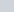$\triangle$x is uncertainty in the specification of position and$\triangle$p is the uncertainty in specification of momentum of an electron, then the product$\triangle$x and$\triangle$p is of the order of ћ such that ћ
(a)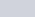$h/2\pi$
(b)$2h/\pi$
(c) 10-19
(d) 10-31

Ans : (a)$h/2\pi$

Q.35: Photoemission with visible light is possible in
(a) rubidium
(b) cesium
(c) zinc
(d) Both (a) and (b)

Ans : (d) Both (a) and (b)

Q.36: Trajectory of an a-particle is shown in the figure below, then impact parameter is

(a) a
(b) b
(c) c
(d) d

Ans : (b) b

Q.37: The ratio of kinetic energy to the total energy of an electron in a Bohr orbit of the hydrogen atom, is
(a) 2 : – 1
(b) 1 : – 1
(c) 1 : 1
(d) 1 : – 2

Ans : (b) 1 : – 1

Q.38: A 12.75 eV electron beam is used to bombard gaseous hydrogen at room temperature, then wavelengths emitted are in
(a) either Paschen, Lyman or Balmer series
(b) either Brackett, Pfund or Lyman series
(c) either Paschen, Brackett or Balmer series
(d) either Lyman, Balmer or Brackett series

Ans : (a) either Paschen, Lyman or Balmer series

Q.39: Isotopes of an atom
(a) have different electronic structure
(b) does not occupy same place in periodic table
(c) contains same number of protons
(d) have same number of neutrons

Ans : (c) contains same number of protons

Q.40: Nuclear fusion is common to the pair
I. uranium based reactor
II. hydrogen bomb
III. energy production in sun
IV. atom bomb
(a) Both I and II
(b) Both II and III
(c) Both III and IV
(d) Both II and IV

Ans : (b) Both II and III

Q.41: In solid state semiconductors, the number and the direction of the flow of charge carriers can be altered by
(a) illuminating light
(b) supplying heat
(c) varying applied voltage
(d) All of the above

Ans : (d) All of the above

Q.42: In the circuit shown, the voltage difference between A and B, if the diode forward voltage drop is 0.3V is

(a) 1.3 V
(b) 2.3 V
(c) 0
(d) 0.5 V

Ans : (b) 2.3 V

Q.43: In a common emitter amplifier circuit using an n-p-n transistor, the phase difference between the input and the output voltages will be
(a) 90°
(b) 135°
(c) 180°
(d) 45°

Ans : (c) 180°

Q.44: LED’s are not used for room lighting (although they are used for automobile bulbs and in industrial lighting) because
(a) our eyes are not comfortable with very intense light
(b) our eyes are not comfortable with monochromatic light
(c) LED’s are much costlier than bulbs tubelights and CFL’s
(d) LED manufacture in mass production will be a very polluting process

Ans : (b) our eyes are not comfortable with monochromatic light

Q.45: A modern communication system acts as a
(a) transmitter
(c) messenger
(d) None of these

Ans : (c) messenger

Q.46: A ground receiver in line-of-sight communication cannot receive direct waves due to
(a) its low frequency
(b) curvature of earth
(c) its high intensity
(d) smaller antenna

Ans : (b) curvature of earth

Q.47: Radio waves are propagated through
(a) ground wave
(b) sky wave
(c) space wave
(d) All of these

Ans : (d) All of these

Q.48: Two field lines can never cross each other because
(a) field lines are closed curves
(b) field lines repels each other
(c) field lines crowded only near the charge
(d) field has a unique direction at each point

Ans : (d) field has a unique direction at each point

Q.49: A singal spreads over a range of frequencies called
(a) signal bandwidth
(b) signal baseband
(c) signal audio frequency
(d) signal video frequency

Ans : (a) signal bandwidth

Q.50: A concave mirror for face viewing has focal length of 0.4 m. The distance at which you hold the mirror from your face in order to see your image upright with a magnification of 5 is
(a) 0.16 m
(b) 1.60 m
(c) 0.32 m
(d) 0.24 m